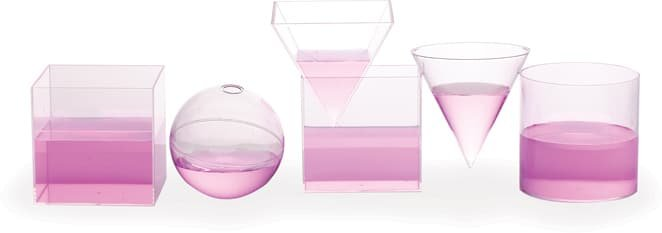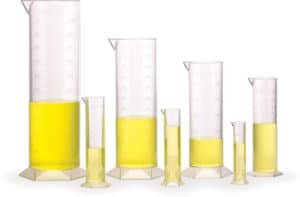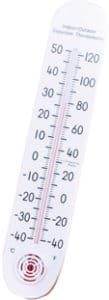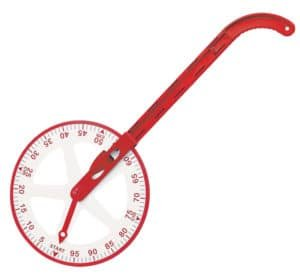# Measurement Materials

#### Linear Measurement

– ruler, metre rule
– tape (measure), windup tape measure
– trundle wheel

#### Time

– analog and digital clocks
– elapsed time ruler

#### Mass

– balances, stacking masses, kitchen scale, spring scale

– measuring grid

#### Volume

– measuring spoons, cups and jugs
– 1cm cubes

– protractors

#### Temperature

– thermometers## Typically measurement is used to answer three questions.Using Length As An Example:

• Which is larger (longer, heavier…..)?
• How large?
• How much larger?

#### When Leanring To Measure, students:

• Become aware of the attribute to measure eg length, area, volume, mass, capacity, time, angle…
• Learn to seriate, that is, to put items in order according to the attribute eg long longer longest.
• Measure things by comparison with a unit eg cm, litres, minutes (any product or material with a calibrated scale).
• Develop estimation about the size of things (Cubic Metre Set).
• Use formulae to calculate the size of things given some measurements (prism, pyramids, Archimedes Measure).

### Mathematical Language

Area, capacity, heavier/lighter, length, longer/shorter, mass, various units, volume.

### Using Measurement Materials

When asked to measure, students must realise what attribute to measure, eg length, mass, etc, choose an appropriate tool and then read the calibrations on the tool. When reading the calibration, an estimation might be required if the measurement falls between two marks on the calibrated scale. Finally the student will need to write the measurement and the appropriate unit down.

### Typical Classroom Requirements

#### A class set:

Each student will need a ruler.

Other measurement materials such as measuring tapes may be shared among two or fourstudents. Students can work in groups of four when using scales, graduated cylinders and trundle wheels. Alternatively, if working in small groups with students rotating through various task centres less measurement material would be required.

Measuring materials are often grouped into themed kits e.g. Liquid Volume Measurement Set.